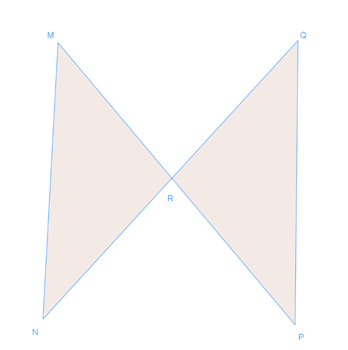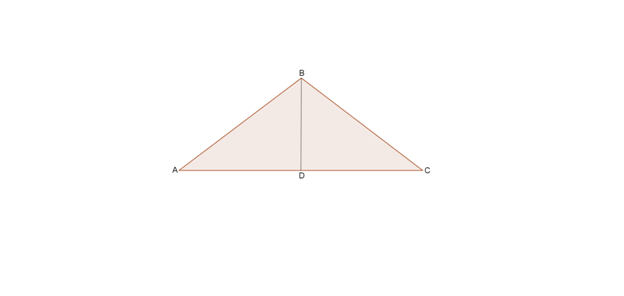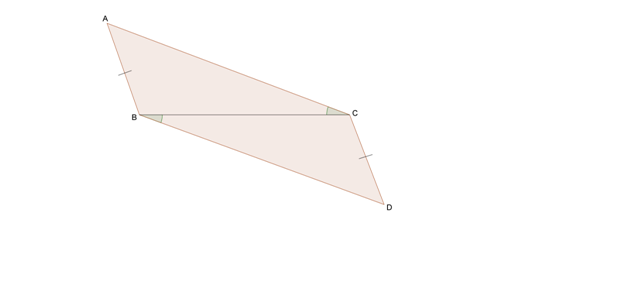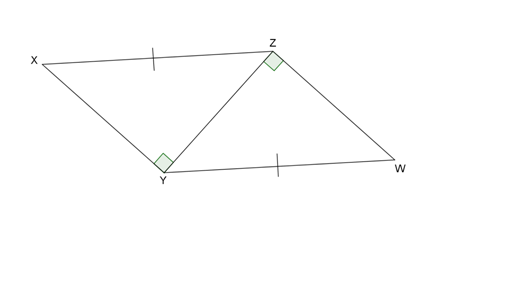# Triangles congruent by SAS and HL proofs

### Triangles congruent by SAS and HL proofs

#### Lessons

• Introduction
a)
1. Similar tirangles VS. Congruent triangles
2. Ways to prove congruency:
• SSS
• SAS
• ASA
• AAS

• 1.
Write a two-column proof.
a)
Given: R is the midpoint of $\overline {MP}$.
R is the midpoint of $\overline {NQ}$.
Prove: $\triangle MRN$$\cong$$\triangle PRQ$b)
Special case for right triangle:
Given: $\triangle ABC$ is an isosceles triangle.
D is the midpoint of $\overline {AC}$.
Prove: $\triangle ABD$$\cong$$\triangle CBD$.• 2.
Write a flow proof
a)
Given: R is the midpoint of $\overline {MP}$.
R is the midpoint of $\overline {NQ}$.
Prove: $\triangle MRN$$\cong$$\triangle PRQ$b)
Special case for right triangle:
Given: $\triangle ABC$ is an isosceles triangle.
D is the midpoint of $\overline {AC}$.
Prove: $\triangle ABD$$\cong$$\triangle CBD$.• 3.
Is there enough information given to prove the following triangles are congruent? If so, explain your reasoning and point out the theorem or postulate you use.
a)b)